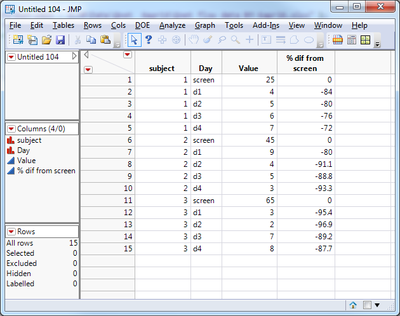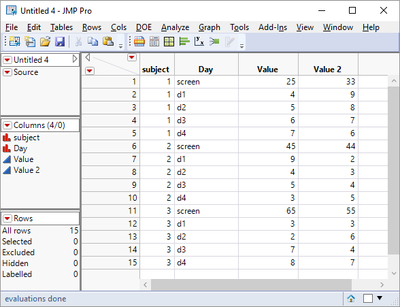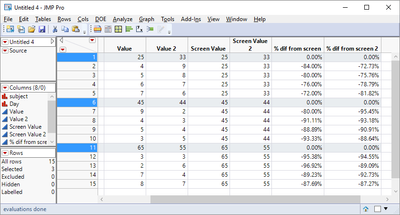Our World Statistics Day conversations have been a great reminder of how much statistics can inform our lives. Do you have an example of how statistics has made a difference in your life? Share your story with the Community!
Choose Language Hide Translation Bar
Highlighted

## How to calculate percentage differences between rows referenced to a cell in another column

Hello all,

I have the following table.  For each subject, I would like to calculate the % difference of the d1, d2, d3 and d4 values in the Value column relative to the screen value.  The formula is ((d1-screen)/screen)*100, repeating for the d2 through d4 values for subject 1.  Then when the subject number changes, the screen value for subject 2 is referenced for the calculation using subject 2's d1 through d4 values, etc.  I am sure there is a way to do this but I have not been successful in identifying one.

Floyd Fox2 ACCEPTED SOLUTIONS

Accepted Solutions
Highlighted

## Re: How to script % differences between rows referenced to a cell in another column

Here is a simple script that does what you want:

Names Default To Here( 1 );

dt = Current Data Table();

// Create a data table with just screen values

dt << select where( :day == "screen" );

dtscreen = dt << subset( selected rows(1 ), selected columns(0 ) );

dtscreen << delete columns( "day" );

dtscreen:Value << set name( "Screen Value" );

// Combine the data back together

dt << Update( With( dtscreen ), Match Columns(:subject = :subject ) );

Close( dtscreen, nosave );

// Make the final calculation

dt << New Column( "% dif from screen", formula((:Value - :screen value) / :Screen Value ), Format( "Percent", 7, 2 ));

Jim
Highlighted

## Re: How to script % differences between rows referenced to a cell in another column

Here's two more aways to do it. Both use the function Col Min() for finding the first row for each subject, and the thus depends on current sorting (Jim's solution has the advantage of being independent of sorting).

dt = Current Data Table();

// Set static values

col1 = dt << New Column("Screen Value", numeric);

col2 = dt << New Column("% dif from screen", numeric, Format(percent, 1));

For Each Row(

col1[] = :Value[Col Min(Row(), :Subject)];

col2[] = (:Value - col1[]) / col1[];

);

// Column formula, all in one step.

dt << New Column("% dif from screen",

Format(percent, 1),

formula((:Value - :Value[Col Min(Row(), :Subject)]) / :Value[Col Min(Row(), :Subject)])

);

6 REPLIES 6
Highlighted

## Re: How to script % differences between rows referenced to a cell in another column

Here is a simple script that does what you want:

Names Default To Here( 1 );

dt = Current Data Table();

// Create a data table with just screen values

dt << select where( :day == "screen" );

dtscreen = dt << subset( selected rows(1 ), selected columns(0 ) );

dtscreen << delete columns( "day" );

dtscreen:Value << set name( "Screen Value" );

// Combine the data back together

dt << Update( With( dtscreen ), Match Columns(:subject = :subject ) );

Close( dtscreen, nosave );

// Make the final calculation

dt << New Column( "% dif from screen", formula((:Value - :screen value) / :Screen Value ), Format( "Percent", 7, 2 ));

Jim
Highlighted

## Re: How to script % differences between rows referenced to a cell in another column

Jim,

Thank you very much this is very usefull.  If I have two (or more) value columns representing different sets of observation on the screen d1, d2 etc, what changes would I have to make in order to calculate the difference for each value column.  Thank you

Floyd

Highlighted

## Re: How to script % differences between rows referenced to a cell in another column

If I am interpreting your expansion request correctly, your data table would now look like this?Using the method that I originally proposed, the script would have a couple of minor changes:

Names Default To Here( 1 );

dt = Current Data Table();

// Create a data table with just screen values

dt << select where( :day == "screen" );

dtscreen = dt << subset( selected rows(1 ), selected columns(0 ) );

dtscreen << delete columns( "day" );

dtscreen:Value << set name( "Screen Value" );

dtscreen:Value 2 << set name( "Screen Value 2" );

// Combine the data back together

dt << Update( With( dtscreen ), Match Columns(:subject = :subject ) );

Close( dtscreen, nosave );

// Make the final calculation

dt << New Column( "% dif from screen", formula((:Value - :screen value) / :Screen Value ), Format( "Percent", 7, 2 ));

dt << New Column( "% dif from screen 2", formula((:Value 2 - :screen value 2) / :Screen Value 2 ), Format( "Percent", 7, 2 ));

This would give you a final table looking like:There is an advantage in using the methodology that MS proposed, in that it can be accomplished just by specifying a formula for the new column.  However, it does rely on determining the Screen row position , which may not be a valid assumption when you are using this with real data.

Jim
Highlighted

## Re: How to script % differences between rows referenced to a cell in another column

Yes, Screen row position may be fiddled with. But if Screen is bound to always have the maximum value within a subject, this formula works too:

:Value / Col Max(:Value, :Subject) - 1

Highlighted

## Re: How to script % differences between rows referenced to a cell in another column

Here's two more aways to do it. Both use the function Col Min() for finding the first row for each subject, and the thus depends on current sorting (Jim's solution has the advantage of being independent of sorting).

dt = Current Data Table();

// Set static values

col1 = dt << New Column("Screen Value", numeric);

col2 = dt << New Column("% dif from screen", numeric, Format(percent, 1));

For Each Row(

col1[] = :Value[Col Min(Row(), :Subject)];

col2[] = (:Value - col1[]) / col1[];

);

// Column formula, all in one step.

dt << New Column("% dif from screen",

Format(percent, 1),

formula((:Value - :Value[Col Min(Row(), :Subject)]) / :Value[Col Min(Row(), :Subject)])

);

Highlighted

## Re: How to script % differences between rows referenced to a cell in another column

MS,

Thank you very much.  I have asked Jim a question and I would pose the same to you.

If I have two (or more) value columns representing different sets of observation on the screen d1, d2 etc, what changes would I have to make in order to calculate the difference for each value column.  Thank you

Floyd

Article Labels

There are no labels assigned to this post.